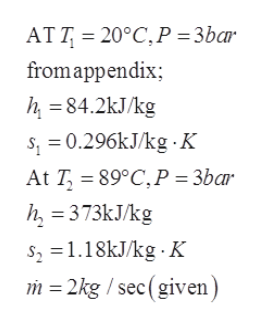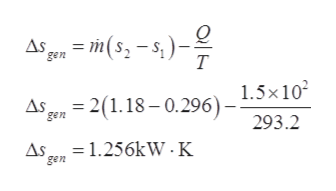# 1) Given 753.06 kW electrical power supplied to a boiler when the temperature of the entering water is 20 C and the exiting temperature is 89 C. The flow of the pressured water is 2 Kg/s. There is a negligible pressure drop through this boiler and it operates at a constant pressure of 3 bars. The specific heat is c = 4,370 J/(Kg K). There is a 1.5(10^5) W rate of heat loss from the boiler during this process to a surrounding at 293.2 k.Answer the following:a) Calculate the exergy destroyed in the process below. The exergy of the fuel entering this process is 51.82 MJ/Kg. The dead state temperature is 293.2 K and pressure is 1 bar. The products of combustion leave this process at the dead state.Process: 0.015 kg is the mass flowrate of fuel (natural gas, CH4) required to heat the water flow to the conditions of the problem above. if the electrical heating device is replaced with a gas fired boiler. The high heating value (HHV) of the fuel is 50.02 MJ/kg.b) The utility providing the electricity to the boiler in problem 1 uses the same fuel as that used in the process above and has an efficiency of 0.35. The cost of the electric boiler is, \$3,000 with a 15 year lifetime. The cost of the fuel based boiler system is \$5000 and has a lifetime of 20 years. The fuel cost is \$0.10/kg and the cost of electricity is \$0.20/kWh. There is a tax charge of \$0.50/kg of CO2 emission that is passed on to the user whether the utility based electrical system or gas fired system is used. The unit is used for 10 hours per day, 280 days per year. Which process would you recommend in terms of fuel consumption, exergy production, carbon dioxide emission and thermoeconomic cost? There is no cost associated with the water input. Note the total amount of fuel consumed is directly related to the carbon dioxide emission from each process.

Question
9 views

1) Given 753.06 kW electrical power supplied to a boiler when the temperature of the entering water is 20 C and the exiting temperature is 89 C. The flow of the pressured water is 2 Kg/s. There is a negligible pressure drop through this boiler and it operates at a constant pressure of 3 bars. The specific heat is c = 4,370 J/(Kg K). There is a 1.5(10^5) W rate of heat loss from the boiler during this process to a surrounding at 293.2 k.

a) Calculate the exergy destroyed in the process below. The exergy of the fuel entering this process is 51.82 MJ/Kg. The dead state temperature is 293.2 K and pressure is 1 bar. The products of combustion leave this process at the dead state.

Process: 0.015 kg is the mass flowrate of fuel (natural gas, CH4) required to heat the water flow to the conditions of the problem above. if the electrical heating device is replaced with a gas fired boiler. The high heating value (HHV) of the fuel is 50.02 MJ/kg.

b) The utility providing the electricity to the boiler in problem 1 uses the same fuel as that used in the process above and has an efficiency of 0.35. The cost of the electric boiler is, \$3,000 with a 15 year lifetime. The cost of the fuel based boiler system is \$5000 and has a lifetime of 20 years. The fuel cost is \$0.10/kg and the cost of electricity is \$0.20/kWh. There is a tax charge of \$0.50/kg of CO2 emission that is passed on to the user whether the utility based electrical system or gas fired system is used. The unit is used for 10 hours per day, 280 days per year. Which process would you recommend in terms of fuel consumption, exergy production, carbon dioxide emission and thermoeconomic cost? There is no cost associated with the water input. Note the total amount of fuel consumed is directly related to the carbon dioxide emission from each process.

check_circle

Step 1

Note:

We are authorized to answer one question at a time, since you have asked three question at time. So, we are answering the first one, Please, re post your question separately for the remaining questions.

Step 2

Entropy and enthalpy,help_outlineImage Transcriptionclose, = 20°C,P = 3bar AT T fromappendix; h =84.2kJ/kg s; = 0.296KJ/kg -K At T, = 89°C,P = 3bar h, = 373kJ/kg s, =1.18KJ/kg · K m = 2kg / sec(given) fullscreen
Step 3

Entropy gener...help_outlineImage TranscriptioncloseAs gen = m( s2 – s, )- 1.5x10 = 2(1.18 – 0.296) – gen 293.2 = 1.256kW · K gen fullscreen

### Want to see the full answer?

See Solution

#### Want to see this answer and more?

Solutions are written by subject experts who are available 24/7. Questions are typically answered within 1 hour.*

See Solution
*Response times may vary by subject and question.
Tagged in

### Thermodynamics